Function Repository Resource:

# ListGrowthPlot

Plot the growth of lists and temporal data

Contributed by: Mark Greenberg
 ResourceFunction["ListGrowthPlot"][{n1,n2,…}] plots the percentage growth of n. ResourceFunction["ListGrowthPlot"][{n11,n12,…},{n21,n22,…},…] plots the percentage growth of each list of n. ResourceFunction["ListGrowthPlot"][td] plots the percentage growth of TemporalData td.

## Details and Options

If td is a TemporalData object with more than one path, ResourceFunction["ListGrowthPlot"][td] plots all paths.
ResourceFunction["ListGrowthPlot"] takes the same options as ListLinePlot and DateListPlot with the following additions and changes:
 AspectRatio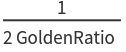ratio of height to width "BalancePoint" 1 zero growth point on the x-axis for all lists Frame None whether to put a frame around the plot InterpolationOrder 2 polynomial degree of curves used in joining data points PlotRange Automatic this setting is overridden and has no effect Ticks Automatic this setting is overridden and has no effect

## Examples

### Basic Examples (2)

Plot the percentage growth of a list of numbers:

 In:=Out=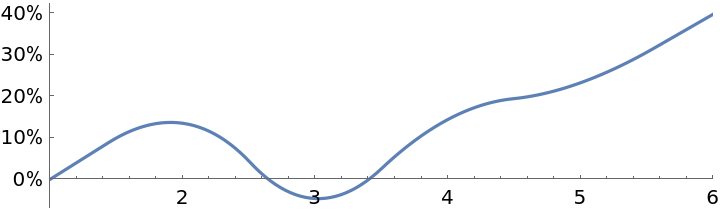Plot the percentage growth of multiple lists of numbers:

 In:=Out=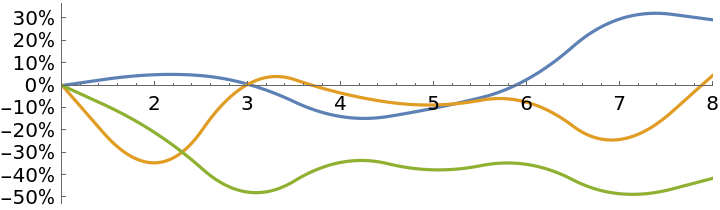### Scope (2)

Plot the percentage growth of a time series:

 In:=Out=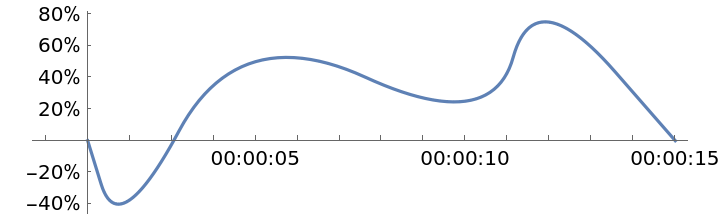Plot the percentage growth of multiple paths within one TemporalData object:

 In:=Out=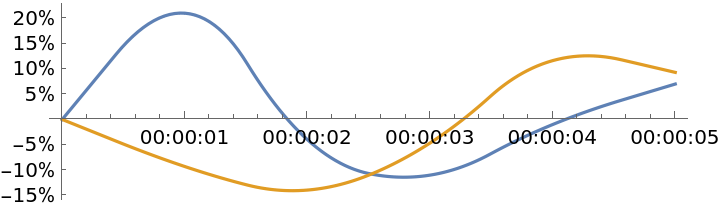### Options (1)

#### BalancePoint (1)

All plotted lines meet at {b, 0} where b is the value of the option "BalancePoint":

 In:=Out=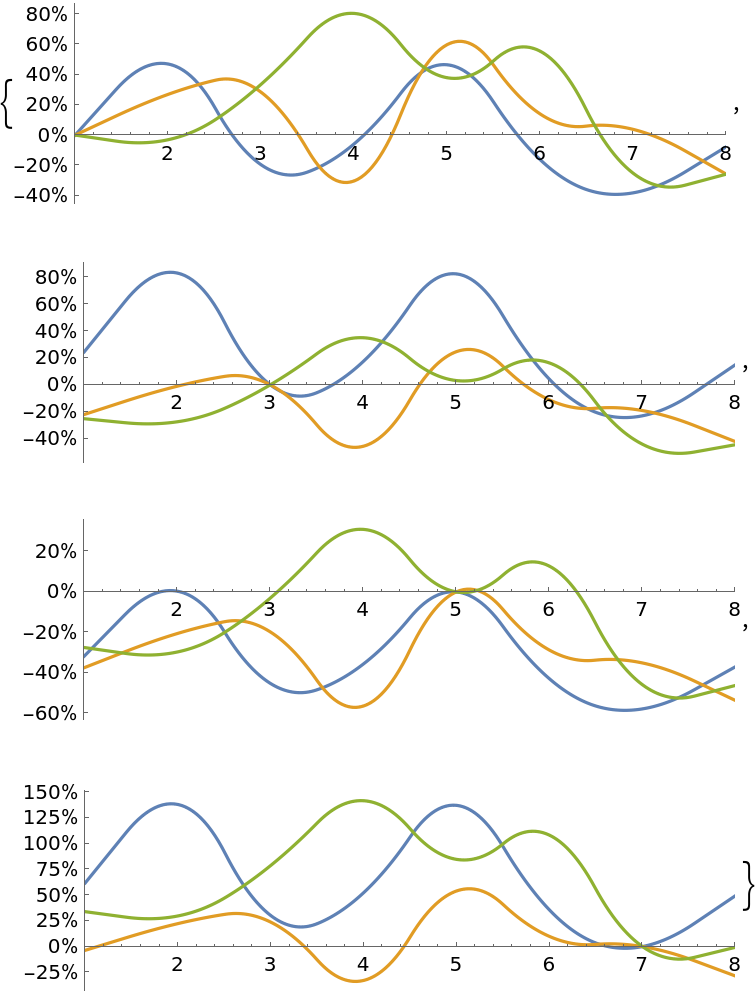### Applications (2)

Plot the performance of two stock investments, even though the stock prices are different:

 In:=Out=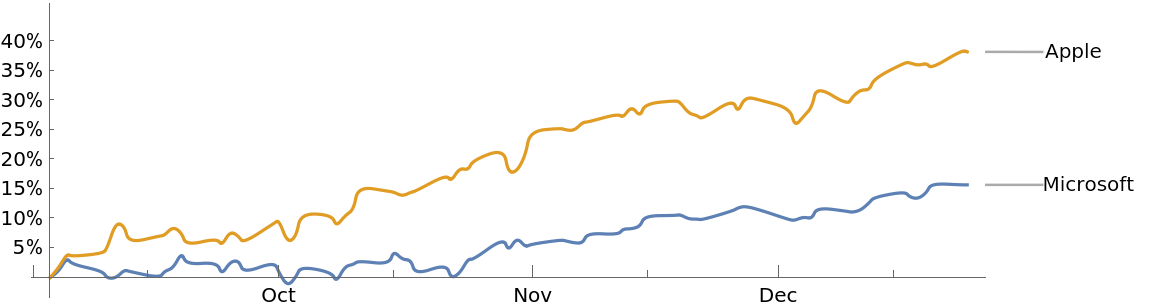Compare the changes in population of two countries, even though the populations are of vastly different magnitude:

 In:=Out=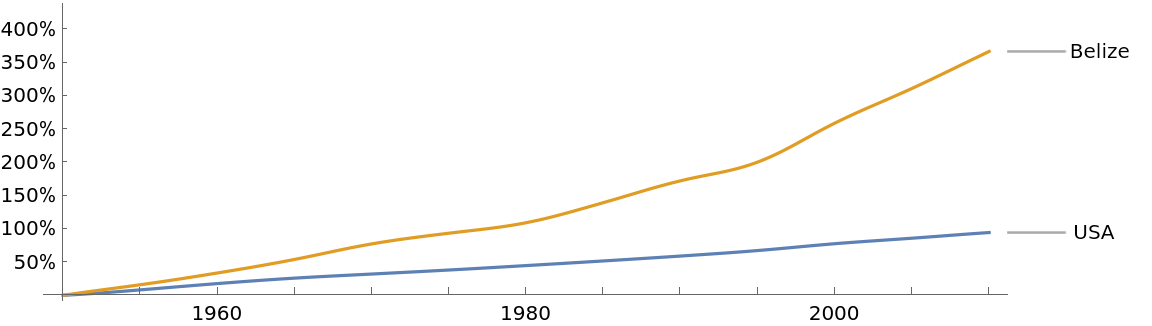### Possible Issues (1)

Percentage change from zero is meaningless, so an error occurs if the value of the balance point is zero for any of the lines plotted:

 In:=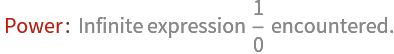Out=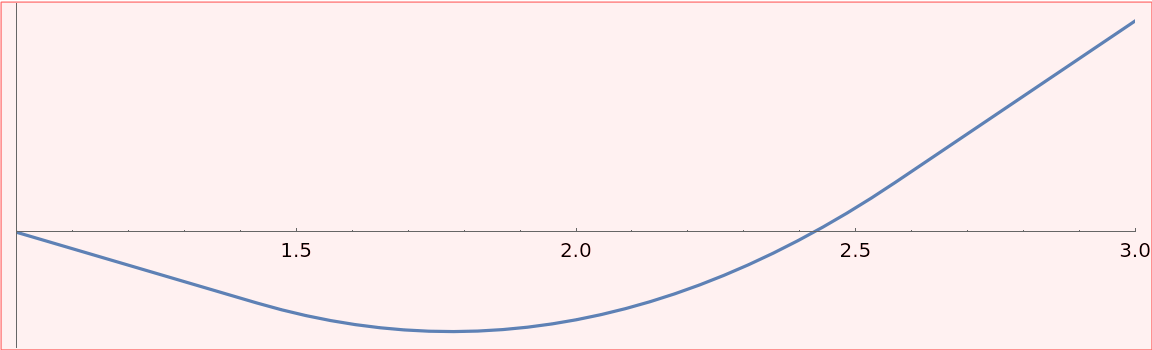Mark Greenberg

## Version History

• 1.0.0 – 04 March 2020